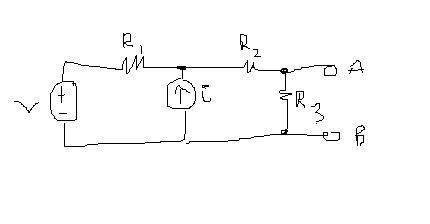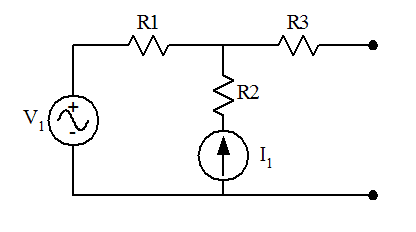# When can I use source transformation?

• TheBlueDot

## Homework Statement

I'm asking this question because I was trying to apply the method to a Thevenin Eq problem and the answer came out wrong.

Also one more related question. According to the textbook, when a current source is connected both in series with a resistor and in parallel with another resistor, we can just remove the resistor that is connected to the current source and carry out the transformation. Is this true always or are there exceptions to this?

I=V/R

## The Attempt at a Solution

1) I transformed the voltage source+ R1 into a current source connected to R1 in parallel.
2) I combined (R3+R2)||R1 and summed up the two current sources and find Rth and Vth.

Thanks!we can just remove the resistor that is connected to the current source and carry out the transformation.
No resistor is removed from the circuit. Current source + parallel resistance is replaced with a voltage source + series resistance. Everything else is kept as it is.

Last edited:
Cnh is correct!If my memory serves me right, when you want to do source transformation, you always want to consider Ohm's Law Vst=Ist*R (where st can be source transformation).
You are correct about R3 being in series with R2, but the combination isn't parallel with R1 because you still have that current source in the middle. You can do a source transformation on the far left side first before combining resistors. You will find doing that first will make it a whole lot easier!

but the combination isn't parallel with R1 because you still have that current source in the middle
Actually, it is. R1 and the series equivalent of R2 and R3 are connected between the same two nodes. Current sources are in parallel with R1. Hence, they can be summed up.
Edit: I see you've mentioned the voltage source transformation, but the OP has done it in step 1 in the attempt at solution...

Last edited:
•SmallDot
Actually, it is. R1 and the series equivalent of R2 and R3 are connected between the same two nodes. Current sources are in parallel with R1. Hence, they can be summed up.
Edit: I see you've mentioned the voltage source transformation, but the OP has done it in step 1 in the attempt at solution...
Right..so once the voltage source is transformed into a current source with R1 in parallel, the current sources can be added together :)

Right..so once the voltage source is transformed into a current source with R1 in parallel, the current sources can be added together :)
Yes.

Also one more related question. According to the textbook, when a current source is connected both in series with a resistor and in parallel with another resistor, we can just remove the resistor that is connected to the current source and carry out the transformation. Is this true always or are there exceptions to this?
This is true. No matter what, an ideal current source will force its specified current through any series-connected resistance in its path. So a resistance in series with a current source has no effect on the current in that branch. You can safely ignore (remove) that resistance when analyzing the overall behavior of the circuit.

For example, in the following circuit R2 can be ignored:The only possible exception that I can think of would be if the particular resistor was being used as a sensor of some sort, perhaps using the potential drop across it to monitor the health of the current source.

•SmallDot and cnh1995
Cnh is correct!If my memory serves me right, when you want to do source transformation, you always want to consider Ohm's Law Vst=Ist*R (where st can be source transformation).
You are correct about R3 being in series with R2, but the combination isn't parallel with R1 because you still have that current source in the middle. You can do a source transformation on the far left side first before combining resistors. You will find doing that first will make it a whole lot easier!
@Aristotle,
Could you please work this problem out? I took the attempt and still didn't get the correct answer. I got this problem from Youtube and the person used node-voltage method instead of source transformation. I appreciate your help!

<

This is true. No matter what, an ideal current source will force its specified current through any series-connected resistance in its path. So a resistance in series with a current source has no effect on the current in that branch. You can safely ignore (remove) that resistance when analyzing the overall behavior of the circuit.

For example, in the following circuit R2 can be ignored:

View attachment 102215

The only possible exception that I can think of would be if the particular resistor was being used as a sensor of some sort, perhaps using the potential drop across it to monitor the health of the current source.